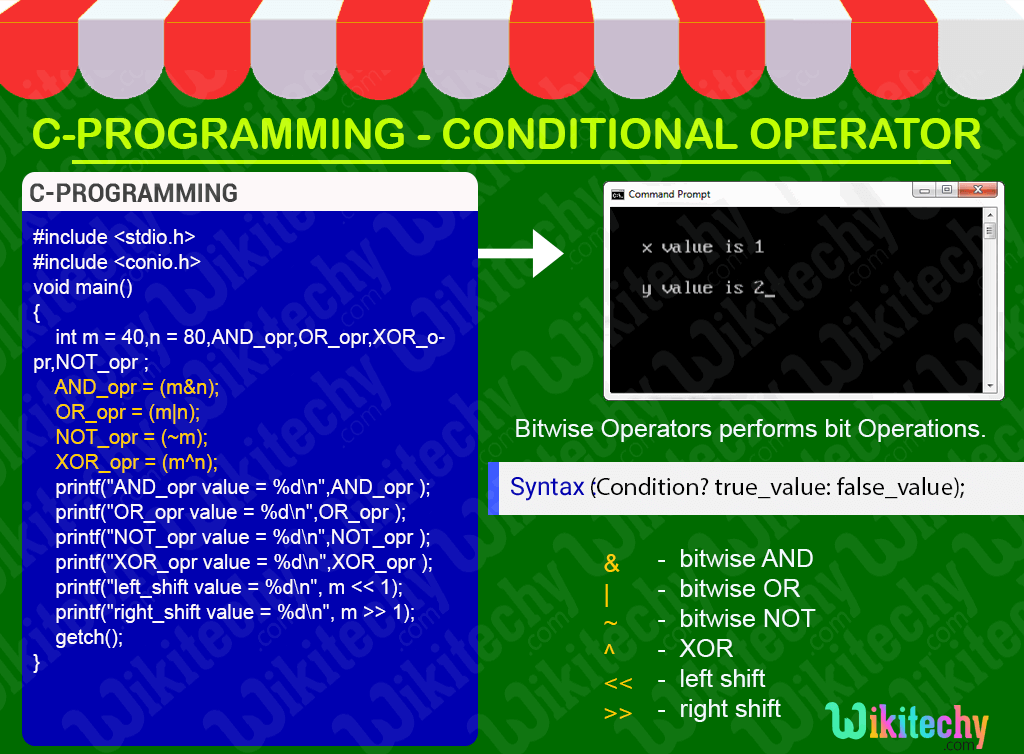# C - Conditional OperatorLearn C - C tutorial - C conditional operator - C examples - C programs

## C Conditional Operator - Definition and Usage

• In C-programming the conditional operator return one value if condition is true and returns another value if condition is false.
• Conditional operators is also called as ternary operator.## C Code - Explanation1. In this statement we declare the variable “x=1” and y as integer.
2. In this statement we check the condition using ternary operator that is if “x==1” means it sets “true” ,so the value for “y” is “2” otherwise it sets the “y” value as “0”.
3. Here we print the value of “x” using the printf statement.
4. Here we print the value of “y” using the printf statement.

## Sample Output - Programming Examples1. Here we printed the value of “x” as “1”.
2. Here in this output the conditional operation has been performed and the condition has been satisfied so the value of the “Y” variable will be printed as “2”.